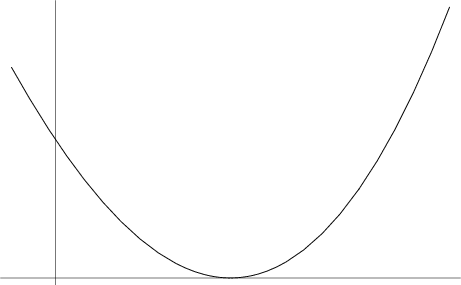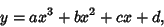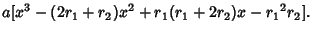## Cubical Parabolic HyperbolaAn equation of the formwhere two of the Roots of the equation coincide (and all three are therefore real), i.e.,See also Cubical Conic Section, Cubical Ellipse, Cubical Hyperbola, Cubical Parabola, Hyperbola